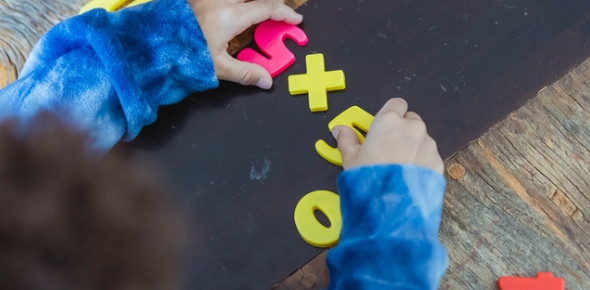# Hard Multiplication Problems With Answers

15 Questions | Attempts: 2022
ShareSettingsHow easily can you multiply various numbers and solve hard multiplication problems? The below quiz contains some hard multiplication questions with answers. So, if you think you have mastered the basic multiplication facts, you really should play it. It will clear out all your doubts regarding your skills. Beginners do not try this quiz as the test gets more difficult after every question. Still, if you think you should give this quiz a try, go ahead then. Best of luck, guys.

• 1.
The product of greatest 2-digit number and smallest 4-digit number is-
• A.

9,900

• B.

99,000

• C.

9,000

• D.

9,900

• 2.
12 + 12 + 12 + 12 + 12 + 12 can be written as-
• A.

12 X 12

• B.

12 + 5

• C.

5 + 12

• D.

5 X 12

• 3.
A box contains 100 toffees. How many toffees are there in 10 such boxes?
• A.

1,100

• B.

100

• C.

1000

• D.

10,000

• 4.
Number of days in 8 weeks are-
• A.

64

• B.

56

• C.

65

• D.

15

• 5.
Which digit should be placed in the empty box to make the multiplication correct?
• A.

3

• B.

4

• C.

5

• D.

6

• 6.
What is four, "nine times"?
• A.

24

• B.

32

• C.

36

• D.

45

• 7.
What is the value of a bee, a tortoise and a group of shells?
• A.

7,   6,   8

• B.

8,   6,   7

• C.

6,  7,   8

• D.

7,   8,   6

• 8.
564 X  ________  X 35  =  35 X 47 X _______  ( Find the missing numbers in the blank).
• A.

546   and   74

• B.

564    and    47

• C.

47    and    35

• D.

47   and   564

• 9.
The product of the place values of beads at ones and thousands place is-
• A.

6000

• B.

5000

• C.

10,000

• D.

3002

• 10.
How many arms do 1000 star fish have?
• A.

500

• B.

5,000

• C.

5,100

• D.

50,000

• 11.
1.25*8 is equal to
• A.

10

• B.

12.5

• C.

100

• D.

1000

• 12.
175*2.3 is equal to
• A.

425.5

• B.

400.5

• C.

402.5

• D.

420.5

• 13.
Twenty-four folders each have 56 sheets of paper inside them. How many sheets of paper are there altogether?
• A.

1344

• B.

3

• C.

1345

• D.

1455

• 14.
A book costs \$ 67. How much will be paid for 102 more such books?
• A.

\$ 6834

• B.

\$ 6835

• C.

\$ 6836

• D.

\$ 6837

• 15.
The monthly salary of a woman is \$ 2,625. What is her annual income pay?
• A.

\$ 31,530

• B.

\$ 31,500

• C.

\$ 31,550

• D.

None

## Related TopicsBack to top
×

Wait!
Here's an interesting quiz for you.